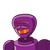# In right angle triangle pqr angle q is equals to 90 degree ,angle r is equals to theta and sin theta is equals to 5/13 then find t

In right angle triangle pqr angle q is equals to 90 degree ,angle r is equals to theta and sin theta is equals to 5/13 then find the values of cos theta and tan theta​

### 1 thought on “In right angle triangle pqr angle q is equals to 90 degree ,angle r is equals to theta and sin theta is equals to 5/13 then find t”

1.Take the given trigonometric ratio as 13k equation (i).

sin θ = 5/13 .. .(i) [Given]

By using the definition write the trigonometric ratio of sin θ and take it as equation

(ii). In right angled ∆PQR, ∠R = θ Let the common multiple be k.

∴ PQ = 5k and PR = 13k

Find QR by using Pythagoras theorem. PR2 = PQ2 + QR2 … [Pythagoras theorem]

∴ (13k)2 = (5k)2 + QR2

∴ 169k2 = 25k2 + QR2

∴ QR2 = 169k2 – 25k2 = 144k2

∴ QR = √(144k2) . . . [Taking square root of both sides] = 12k